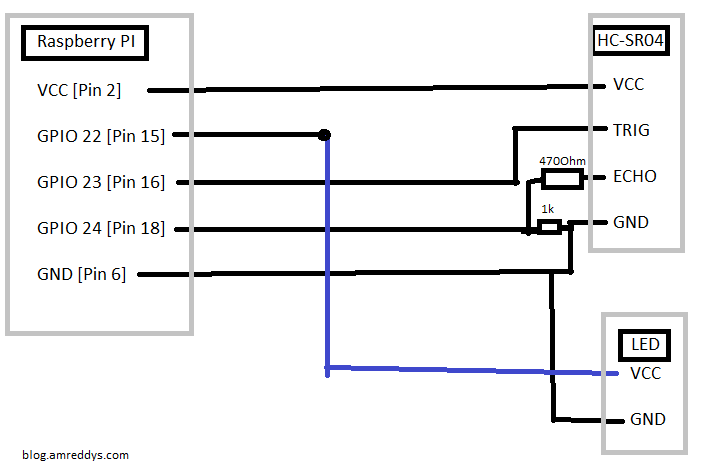Using Raspberry PI B+ for automated distance measurement and alert with LED for a driver alert system

The idea is to alert a driver when something comes too near to his vehicle. In order to prototype this the alert is chosen as an LED and Raspberry Pi B+ with a HC-SR04 to measure the distance. I have followed the tutorial here. I ended up with the following values for the resistors to by pass additional voltage from the echo of HC-SR04 circuit.

R1 = 470 Ohm and R2 = 1K Ohm.

I haven’t used any resistor for the LED because it just runs for a few seconds or at maximum a few minutes during testing but I highly recommend you to use a resistor with LED.

Following is the schematic I’ve followed. Obviously looking bad because I’m new to drawing schematics using Paint.The Python Code is almost identical to the tutorial I’ve followed but I’ve made a few modifications.

1. Added the LED to hold GPIO 22
2. Infinite loop to keep the calculations running all the time and a try catch block to clean GPIO if the program is run manually and halted with Ctrl+C from SSH Console.

import RPi.GPIO as GPIO
import time

GPIO.setmode(GPIO.BCM)

LED = 22
TRIG = 23
ECHO = 24

print "Distance Measurement In Progress"

GPIO.setup(LED,GPIO.OUT)
GPIO.setup(TRIG,GPIO.OUT)
GPIO.setup(ECHO,GPIO.IN)
try:
while(1):
GPIO.output(TRIG, False)
#print "Waiting For Sensor To Settle"
time.sleep(0.1)

GPIO.output(TRIG, True)
time.sleep(0.00001)
GPIO.output(TRIG, False)

while GPIO.input(ECHO)==0:
pulse_start = time.time()

while GPIO.input(ECHO)==1:
pulse_end = time.time()

pulse_duration = pulse_end - pulse_start

distance = pulse_duration * 17150

distance = round(distance, 2)
if distance<10:
GPIO.output(LED,True)
else:
GPIO.output(LED,False)

print "Distance:",distance,"cm"
except KeyboardInterrupt:
GPIO.cleanup()

I don’t want to manually start the program each time raspberry pi boots so I’ve added this script to run at startup by adding the command to /etc/rc.local using the tutorial here

Here are the results

If you weren’t too clear of how to do this on your own head to the tutorials. I’ve mention only the changes and didn’t write the actual tutorial. Hope that helped.

http://www.modmypi.com/blog/hc-sr04-ultrasonic-range-sensor-on-the-raspberry-pi

https://www.raspberrypi.org/documentation/linux/usage/rc-local.md